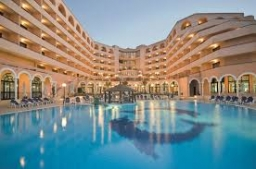# Accommodated 6906

Tourists are accommodated in three hotels. There are eight more tourists in the second hotel than in the first. In the third hotel are 14 more than in the second. How many tourists stayed in each hotel if there were 258 in total?

a =  76
b =  84
c =  98

### Step-by-step explanation:

b = 8 +a
c = 14+b
a+b+c = 258

a-b = -8
b-c = -14
a+b+c = 258

Row 3 - Row 1 → Row 3
a-b = -8
b-c = -14
2b+c = 266

Pivot: Row 2 ↔ Row 3
a-b = -8
2b+c = 266
b-c = -14

Row 3 - 1/2 · Row 2 → Row 3
a-b = -8
2b+c = 266
-1.5c = -147

c = -147/-1.5 = 98
b = 266-c/2 = 266-98/2 = 84
a = -8+b = -8+84 = 76

a = 76
b = 84
c = 98

Our linear equations calculator calculates it.Did you find an error or inaccuracy? Feel free to write us. Thank you!

Tips for related online calculators
Do you have a system of equations and looking for calculator system of linear equations?